# Thevenin’s, Norton’s, and Maximum Power Transfer Theorems

## Network Analysis Techniques

• #### Question 1

Suppose a 12 volt lead-acid battery has an internal resistance of 20 milli-ohms (20 mΩ):If a short-circuit were placed across the terminals of this large battery, the fault current would be quite large: 600 amps!

Now suppose three of these batteries were connected directly in parallel with one another: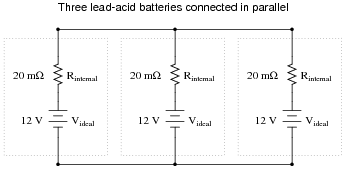Reduce this network of parallel-connected batteries into either a Thévenin or a Norton equivalent circuit, and then re-calculate the fault current available at the terminals of the three-battery “bank” in the event of a direct short-circuit.

• #### Question 2

What would happen if a wire having no resistance at all (0 Ω) were connected directly across the terminals of a 6-volt battery? How much current would result, according to Ohm’s Law?Suppose we were to short-circuit a 6-volt battery in the manner just described and measure 8 amps of current. Why don’t the calculated figures from the previous paragraph agree with the actual measurement?

• #### Question 3

 Don’t just sit there! Build something!!

Learning to mathematically analyze circuits requires much study and practice. Typically, students practice by working through lots of sample problems and checking their answers against those provided by the textbook or the instructor. While this is good, there is a much better way.

You will learn much more by actually building and analyzing real circuits, letting your test equipment provide the “answers” instead of a book or another person. For successful circuit-building exercises, follow these steps:

1. Carefully measure and record all component values prior to circuit construction.
2. Draw the schematic diagram for the circuit to be analyzed.
3. Carefully build this circuit on a breadboard or other convenient medium.
4. Check the accuracy of the circuit’s construction, following each wire to each connection point, and verifying these elements one-by-one on the diagram.
5. Mathematically analyze the circuit, solving for all values of voltage, current, etc.
6. Carefully measure those quantities, to verify the accuracy of your analysis.
7. If there are any substantial errors (greater than a few percent), carefully check your circuit’s construction against the diagram, then carefully re-calculate the values and re-measure.

Avoid very high and very low resistor values, to avoid measurement errors caused by meter “loading”. I recommend resistors between 1 kΩ and 100 kΩ, unless, of course, the purpose of the circuit is to illustrate the effects of meter loading!

One way you can save time and reduce the possibility of error is to begin with a very simple circuit and incrementally add components to increase its complexity after each analysis, rather than building a whole new circuit for each practice problem. Another time-saving technique is to re-use the same components in a variety of different circuit configurations. This way, you won’t have to measure any component’s value more than once.

• #### Question 4

Sometimes you will see amplifier circuits expressed as collections of impedances and dependent sources: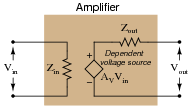With this model, the amplifier appears as a load (Zin) to whatever signal source its input is connected to, boosts that input voltage by the gain factor (AV), then outputs the boosted signal through a series output impedance (Zout) to whatever load is connected to the output terminals: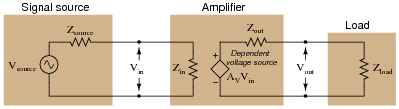Explain why all these impedances (shown as resistors) are significant to us as we seek to apply amplifier circuits to practical applications. Which of these impedances do you suppose are typically easier for us to change, if they require changing at all?

• #### Question 5

The voltage divider network employed to create a DC bias voltage for many transistor amplifier circuits has its own effect on amplifier input impedance. Without considering the presence of the transistor or the emitter resistance, calculate the impedance as “seen” from the input terminal resulting from the two resistors R1 and R2 in the following common-collector amplifier circuit: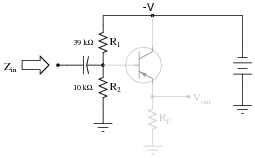Remember, what you are doing here is actually determining the Thévenin/Norton equivalent resistance as seen from the input terminal by an AC signal. The input coupling capacitor reactance is generally small enough to be safely ignored.

Next, calculate the input impedance of the same circuit, this time considering the presence of the transistor and emitter resistor, assuming a current gain (β or hfe) of 60, and the following formula for impedance at the base resulting from β and RE:

 $$Z_B \approx (\beta + 1) R_E$$Develop an equation from the steps you take in calculating this impedance value.

• #### Question 6

Determining the output impedance of a common-emitter amplifier is impossible unless we know how to model the transistor in terms of components whose behavior is simple to express.When in its active mode, a transistor operates like a current regulator. This is similar enough to the behavior of a current source that we may use a source to model the transistor’s behavior for the sake of this impedance determination: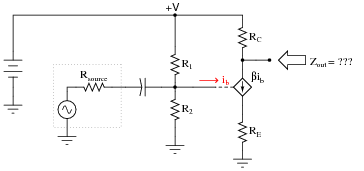Now, apply the same steps you would use in determining the Thévenin or Norton equivalent impedance to the output of this amplifier circuit, and this will yield the amplifier’s output impedance. Draw an equivalent circuit for the amplifier during this Thévenizing/Nortonizing process to show how the output impedance is determined.

• #### Question 7

An electric arc welder is a low-voltage, high-current power source designed to supply enough electric current to sustain an arc capable of welding metal with its high temperature: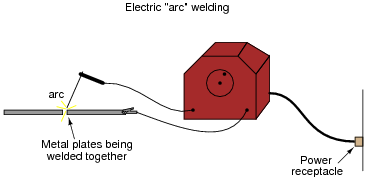It is possible to derive a Norton equivalent circuit for an arc welder based on empirical measurements of voltage and current. Take for example these measurements, under loaded and no-load conditions: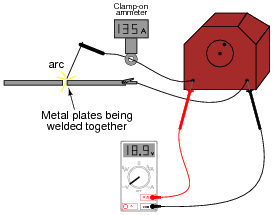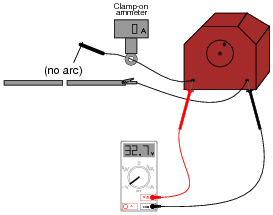Based on these measurements, draw a Norton equivalent circuit for the arc welder.

• #### Question 8

Thévenize this resistive network:• #### Question 9

Resistive voltage dividers are very useful and popular circuits. However, it should be realized that their output voltages ßag” under load: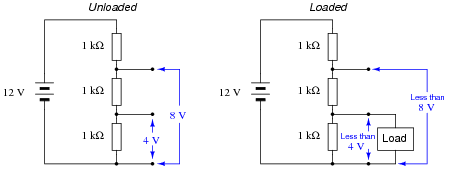Just how much a voltage divider’s output will sag under a given load may be a very important question in some applications. Take for instance the following application where we are using a resistive voltage divider to supply an engine sensor with reduced voltage (8 volts) from the 12 volt battery potential in the automobile: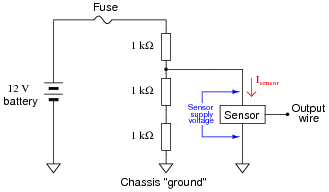If the sensor draws no current (Isensor = 0 mA), then the voltage across the sensor supply terminals will be 8 volts. However, if we were asked to predict the voltage across the sensor supply terminals for a variety of different sensor current conditions, we would be faced with a much more complex problem:

 Sensor current (Isensor) Sensor supply voltage 0 mA 8 volts 1 mA 2 mA 3 mA 4 mA 5 mA

One technique we could use to simplify this problem is to reduce the voltage divider resistor network into a Thévenin equivalent circuit. With the three-resistor divider reduced to a single resistor in series with an equivalent voltage source, the calculations for sensor supply voltage become much simpler.

Show how this could be done, then complete the table of sensor supply voltages shown above.

• #### Question 10

Give a step-by-step procedure for “Thévenizing” any circuit: finding the Thévenin equivalent voltage (VThevenin) and Thévenin equivalent resistance (RThevenin).

Step #1:
Step #2:
• #### Question 11

A voltage source is a source of electricity that (ideally) outputs a constant voltage. That is, a perfect voltage source will hold its output voltage constant regardless of the load imposed upon it:In real life, there is no such thing as a perfect voltage source, but sources having extremely low internal resistance come close.

Another type of electricity source is the current source, which (ideally) outputs a constant current regardless of the load imposed upon it. A common symbol for a current source is a circle with an arrow inside (always pointing in the direction of conventional flow, not electron flow!). Another symbol is two intersecting circles, with an arrow nearby pointing in the direction of conventional flow:Predict how an ideal current source would behave for the following two load scenarios:• #### Question 12

Ideal voltage sources and ideal current sources, while both being sources of electrical power, behave very differently from one another:Explain how each type of electrical source would behave if connected to a variable-resistance load. As this variable resistance were increased and decreased, how would each type of source respond?

• #### Question 13

A very common sort of graph used in electronics work is the load line, showing all possibilities of load voltage and load current that a particular power source is able to supply to a load: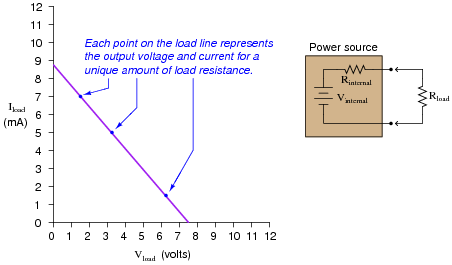Note how the load line shows the voltage “sag” of the power source in relation to the amount of current drawn by the load. At high currents, the output voltage will be very low (upper-left end of load line). At low currents, the output voltage will be near its maximum (lower-right end of load line). If all internal components of the power source are linear in nature, the load line will always be perfectly straight.

Plot the load line for a power source having an internal voltage (Vinternal) of 11 volts and an internal resistance (Rinternal) of 1.2 kΩ. Superimpose your load line onto the load line graph shown above. Hint: it only takes two points to define a line!

• #### Question 14

Give a step-by-step procedure for reducing this circuit to a Norton equivalent circuit (one current source in parallel with one resistor):• #### Question 15

Suppose you had an AC/DC power supply, which performed as follows (open-circuit and loaded test conditions):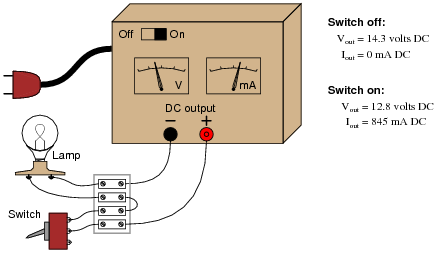Draw a Thévenin equivalent circuit to model the behavior of this power supply.

• #### Question 16

Suppose you were handed a black box with two metal terminals on one side, for attaching electrical (wire) connections. Inside this box, you were told, was a voltage source (an ideal voltage source connected in series with a resistance):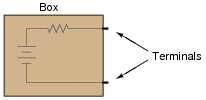How would you experimentally determine the voltage of the ideal voltage source inside this box, and how would you experimentally determine the resistance of the series resistor? By “experimentally,” I mean determine voltage and resistance using actual test equipment rather than assuming certain component values (remember, this “black box” is sealed, so you cannot look inside!).

• #### Question 17

Suppose you were handed a black box with two metal terminals on one side, for attaching electrical (wire) connections. Inside this box, you were told, was a current source (an ideal current source connected in parallel with a resistance):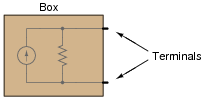How would you experimentally determine the current of the ideal current source inside this box, and how would you experimentally determine the resistance of the parallel resistor? By “experimentally,” I mean determine current and resistance using actual test equipment rather than assuming certain component values (remember, this “black box” is sealed, so you cannot look inside!).

• #### Question 18

Suppose you were handed a black box with two metal terminals on one side, for attaching electrical (wire) connections. Inside this box, you were told, was a voltage source connected in series with a resistance.Your task was to experimentally determine the values of the voltage source and the resistor inside the box, and you did just that. From your experimental data you then sketched a circuit with the following component values: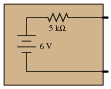However, you later discovered that you had been tricked. Instead of containing a single voltage source and a single resistance, the circuit inside the box actually looked like this: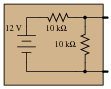Demonstrate that these two different circuits are indistinguishable from the perspective of the two metal terminals, and explain what general principle this equivalence represents.

• #### Question 19

Give a step-by-step procedure for reducing this circuit to a Thévenin equivalent circuit (one voltage source in series with one resistor):• #### Question 20

Suppose you were handed a black box with two metal terminals on one side, for attaching electrical (wire) connections. Inside this box, you were told, was a current source connected in parallel with a resistance.Your task was to experimentally determine the values of the current source and the resistor inside the box, and you did just that. From your experimental data you then sketched a circuit with the following component values: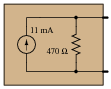However, you later discovered that you had been tricked. Instead of containing a current source and a resistor, the circuit inside the box was actually a voltage source connected in series with a resistor: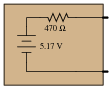Demonstrate that these two different circuits are indistinguishable from the perspective of the two metal terminals, and explain what general principle this equivalence represents.

• #### Question 21

An AC voltage source with an internal (“Thévenin”) resistance of 50 Ω is connected to a step-down transformer with a winding ratio of 10:1. What is the equivalent source voltage and resistance, as seen from the load terminals?• #### Question 22

Calculate the voltage dropped across the load resistor, and the current through the load resistor, for the following load resistance values in this circuit:Rload Eload Iload 1 k Ω 2 k Ω 5 k Ω 8 k Ω 10 k Ω

Do the “boxed” components in this circuit behave more like a constant voltage source, or a constant current source? Explain your answer.

• #### Question 23

Calculate the voltage dropped across the load resistor, and the current through the load resistor, for the following load resistance values in this circuit:Rload Eload Iload 1 k Ω 2 k Ω 5 k Ω 8 k Ω 10 k Ω

Do the “boxed” components in this circuit behave more like a constant voltage source, or a constant current source? Explain your answer.

• #### Question 24

In the following circuit, an adjustable voltage source is connected in series with a resistive load and another voltage source:Determine what will happen to the current in this circuit if the adjustable voltage source is increased.

In this next circuit, an adjustable voltage source is connected in series with a resistive load and a current source:Now determine what will happen to the current in this second circuit if the adjustable voltage source is increased.

One way to define electrical resistance is by comparing the change in applied voltage (∆V) to the change in resultant current (∆I). This is mathematically expressed by the following ratio:

 $$R= \frac{\triangle V}{\triangle I}$$

From the perspective of the adjustable voltage source (Vadjust), and as defined by the above equation, which of these two circuits has the greatest resistance? What does this result suggest about the equivalent resistance of a constant-voltage source versus the equivalent resistance of a constant-current source?

• #### Question 25

A practical current source may be built using a battery and a special semiconductor component known as a current-limiting diode:The current-limiting diode acts as a variable resistance, to regulate current through it at a constant value: if current increases, its resistance increases to reduce the current back to where it should be; if current decreases, its resistance decreases to increase current up to where it should be.

Determine the amount of voltage output by an open-circuited (ideal) current source. Contrast this with the voltage output by the practical current source shown in the diagram. Finally, draw an equivalent circuit showing an ideal current source somehow connected to a resistance in such a way that its open-circuited output voltage is identical to the practical current source.

• #### Question 26

Electrochemical batteries are supposed to act as constant voltage sources, outputting an unchanging voltage for a wide range of load currents. The output voltage of real batteries, though, always “sags” to some degree under the influence of a load.

Explain why this is so, in terms of modeling the battery as an ideal voltage source combined with a resistance. How do you suggest the internal resistance of a chemical battery be experimentally measured?

• #### Question 27

Load lines are special types of graphs used in electronics to characterize the output voltage and current behavior of different power sources:If we know that all the internal components of a power source are inherently linear, we know that the load line plot will indeed by a straight line. And, if we know the plot will be a straight line, all we need in order to plot a complete load line are two data points.

Usually, the easiest data points to gather for a circuit - whether it be a real circuit or an hypothetical circuit existing on paper only - is the open-circuit condition and the short-circuit condition. In other words, we see how much voltage the source will output with no load connected (Iload = 0 milliamps) and then we see how much current the source will output into a direct short (Vload = 0 volts):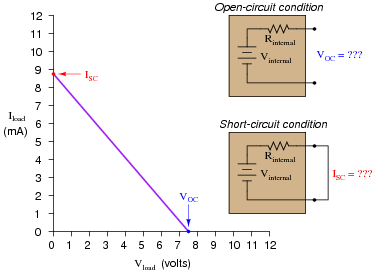Suppose we have two differently-constructed power sources, yet both of these sources share the same open-circuit voltage (VOC) and the same short-circuit current (ISC). Assuming the internal components of both power sources are linear in nature, explain how we would know without doubt that the two power sources were electrically equivalent to one another. In other words, explain how we would know just from the limited data of VOC and ISC that these two power sources will behave exactly the same when connected to the same load resistance, whatever that load resistance may be.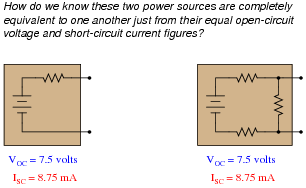• #### Question 28

Suppose you were handed a black box with two metal terminals on one side, for attaching electrical (wire) connections. Inside this box, you were told, was a voltage source connected in series with a resistance.Your task was to experimentally determine the values of the voltage source and the resistor inside the box, and you did just that. From your experimental data you then sketched a circuit with the following component values: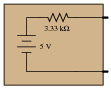However, you later discovered that you had been tricked. Instead of containing a single voltage source and a single resistance, the circuit inside the box actually looked like this: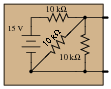Demonstrate that these two different circuits are indistinguishable from the perspective of the two metal terminals, and explain what general principle this equivalence represents.

• #### Question 29

Examine this circuit, consisting of an ideal voltage source and several resistors:First, calculate the voltage seen at the load terminals with a voltmeter directly connected across them (an open-circuit condition):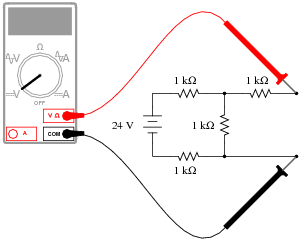Next, calculate the current seen at the load terminals with an ammeter directly connected across them (a short-circuit condition):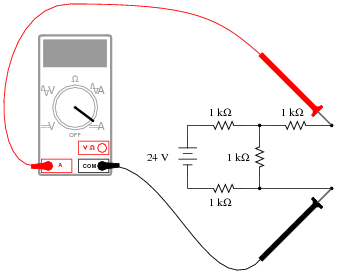Based on these open- and short-circuit calculations, draw a new circuit consisting of a single voltage source and a single (series) resistor that will respond in the exact same manner. In other words, design an equivalent circuit for the circuit shown here, using the minimum number of possible components.

• #### Question 30

An electric arc welder is a low-voltage, high-current power source used to generate hot arcs capable of melting metal. Note the voltage and current measurements taken for this particular welder: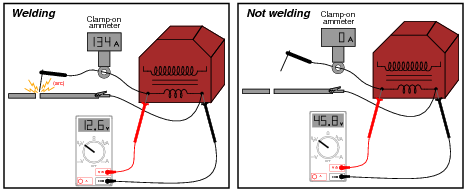Determine two Thévenin equivalent circuits for the arc welder. The first circuit will simply be an AC voltage source and an internal impedance. The second circuit will be a voltage source and internal impedance connected through an ideal transformer with a step-down ratio of 8 to 1:• #### Question 31

An electric arc welder is a low-voltage, high-current power source used to generate hot arcs capable of melting metal. Note the voltage and current measurements taken for this particular welder: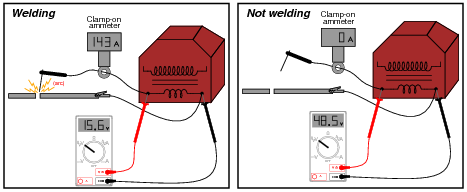Determine two Thévenin equivalent circuits for the arc welder. The first circuit will simply be an AC voltage source and an internal impedance. The second circuit will be a voltage source and internal impedance connected through an ideal transformer with a step-down ratio of 8 to 1:• #### Question 32

Is it possible to regard this complex network of power sources and resistances as a simple voltage source (one ideal voltage source in series with an internal resistance), or as a simple current source (one ideal current source in parallel with an internal resistance)? Why or why not?• #### Question 33

Give a step-by-step procedure for “Nortonizing” any circuit: finding the Norton equivalent current (VNorton) and Norton equivalent resistance (RNorton).

Step #1:
Step #2:
• #### Question 34

Use Thévenin’s Theorem to determine a simple equivalent circuit for the 10 volt source, the 5 kΩ resistor, and the 2.2 kΩ resistor; then calculate the voltage across the 1 kΩ load:This exercise may seem pointless, as it is easy enough to obtain the answer simply by series-parallel analysis of this circuit. However, there is definite value in determining a Thévenin equivalent circuit for the voltage source, 5 kΩ resistor, and 2.2 kΩ resistor if the load voltage for several different values of load resistance needs to be predicted. Explain why Thévenin’s Theorem becomes the more efficient way to predict load voltage if multiple load resistor values are considered.

• #### Question 35

Use Thévenin’s Theorem to determine a simple equivalent circuit for the 3 mA source, the 8 kΩ resistor, and the 3.3 kΩ resistor; then calculate the voltage across the 1 kΩ load:• #### Question 36

Convert the following Thévenin equivalent circuit into a Norton equivalent circuit:• #### Question 37

Convert the following Norton equivalent circuit into a Thévenin equivalent circuit:• #### Question 38

An ideal (perfect) current source is an abstraction with no accurate realization in life. However, we may approximate the behavior of an ideal current source with a high-voltage source and large series resistance:Such a Thevenin equivalent circuit, however imperfect, will maintain a fairly constant current through a wide range of load resistance values.

Similarly, an ideal (perfect) voltage source is an abstraction with no accurate realization in life. Thankfully, though, it is not difficult to build voltage sources that are relatively close to perfect: circuits with very low internal resistance such that the output voltage sags only a little under high-current conditions.

But suppose we lived in a world where things were the opposite: where close-to-ideal current sources were simpler and more plentiful than close-to-ideal voltage sources. Draw a Norton equivalent circuit showing how to approximate an ideal voltage source using an ideal (perfect) current source and a shunt resistance.

• #### Question 39

One day an electronics student decides to build her own variable-voltage power source using a 6-volt battery and a 10 kΩ potentiometer: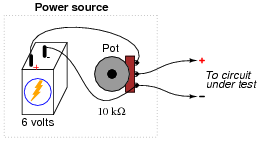She tests her circuit by connecting a voltmeter to the output terminals and verifying that the voltage does indeed increase and decrease as the potentiometer knob is turned.

Later that day, her instructor assigns a quick lab exercise: measure the current through a parallel resistor circuit with an applied voltage of 3 volts, as shown in the following schematic diagram.Calculating current in this circuit is a trivial exercise, she thinks to herself: 3 V ÷ 500 Ω = 6 mA. This will be a great opportunity to use the new power source circuit, as 3 volts is well within the voltage adjustment range!

She first sets up her circuit to output 3 volts precisely (turning the 10 kΩ potentiometer to the 50% position), measuring with her voltmeter as she did when initially testing the circuit. Then she connects the output leads to the two parallel resistors through her multimeter (configured as an ammeter), like this: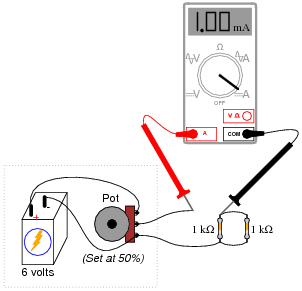However, when she reads her ammeter display, the current only measures 1 mA, not 6 mA as she predicted. This is a very large discrepancy between her prediction and the measured value for current!

Use Thévenin’s Theorem to explain what went wrong in this experiment. Why didn’t her circuit behave as she predicted it would?

• #### Question 40

Observe the following circuit:Note that it is not reducible to a single resistance and power source. In other words, it is not a series-parallel combination circuit. And, while it is a bridge circuit, you are not able to simply analyze the resistor ratios because it is obviously not in a state of balance!

If you were asked to calculate voltage or current for any component in this circuit, it would be a difficult task . . . unless you know either Thévenin’s or Norton’s theorems, that is! Apply either one of these theorems to the determination of voltage across the 2.2 kΩ resistor.

Hint: consider the 2.2 kΩ resistor as the load in a Thévenin or Norton equivalent circuit.

• #### Question 41

Plot the power dissipation of the load resistance, for several values between 1 kΩ and 20 kΩ:• #### Question 42

Explain what the Maximum Power Transfer Theorem is, in your own words.

• #### Question 43

At what load resistance value is the power dissipation maximized for the source’s internal resistance?• #### Question 44

Explain why it is important for optimum performance to connect speakers of the proper impedance to an audio power amplifier.

• #### Question 45

At what source resistance value is the power dissipation maximized for the load?• #### Question 46

Suppose we were planning to use a photovoltaic panel to generate electricity and electrolyze water into hydrogen and oxygen gas: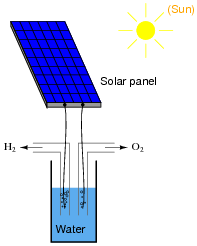Our goal is to electrolyze as much water as possible, and this means we must maximize the electrolysis cell’s power dissipation. Explain how we could experimentally determine the optimum internal resistance of the electrolysis cell, prior to actually building it, using nothing but the solar panel, a rheostat, and a DMM (digital multimeter).